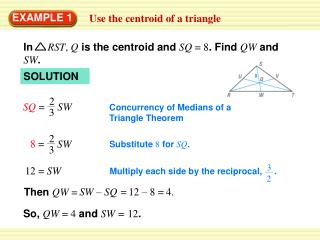# EXAMPLE 1 - PowerPoint PPT PresentationDownload PresentationEXAMPLE 1

Download Presentation## EXAMPLE 1

- - - - - - - - - - - - - - - - - - - - - - - - - - - E N D - - - - - - - - - - - - - - - - - - - - - - - - - - -
##### Presentation Transcript

1. In RST, Qis the centroid and SQ = 8. Find QWand SW. SQ = SW 2 2 3 3 8= SW 3 2 Multiply each side by the reciprocal, . 12= SW 12 – 8 = 4. SW – SQ = Then QW = EXAMPLE 1 Use the centroid of a triangle SOLUTION Concurrency of Medians of a Triangle Theorem Substitute 8 for SQ. So, QW = 4 and SW = 12.

2. Sketch FGH. Then use the Midpoint Formula to find the midpoint Kof FHand sketch median GK. 2 + 6 , 5 + 1 K( ) = 2 2 EXAMPLE 2 Standardized Test Practice SOLUTION K(4, 3) The centroid is two thirds of the distance from each vertex to the midpoint of the opposite side.

3. The distance from vertex G(4, 9)to K(4, 3)is 9–3 = 6 units. So, the centroid is (6) = 4 units down from G on GK. The correct answer is B. 2 3 EXAMPLE 2 Standardized Test Practice The coordinates of the centroid Pare (4, 9 – 4), or (4, 5).

4. 1. If SC = 2100 feet, findPS andPC. ANSWER 700 ft, 1400 ft for Examples 1 and 2 GUIDED PRACTICE There are three paths through a triangular park. Each path goes from the midpoint of one edge to the opposite corner. The paths meet at point P.

5. 2. If BT = 1000 feet, find TC andBC. ANSWER 1000 ft, 2000 ft for Examples 1 and 2 GUIDED PRACTICE There are three paths through a triangular park. Each path goes from the midpoint of one edge to the opposite corner. The paths meet at point P.

6. 3. If PT = 800 feet, findPA andTA. ANSWER 1600 ft, 2400 ft for Examples 1 and 2 GUIDED PRACTICE There are three paths through a triangular park. Each path goes from the midpoint of one edge to the opposite corner. The paths meet at point P.